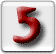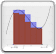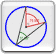5ème
Recueil d'exercices pour les élèves de niveau 5ème Secondaire - HumanitésQuelques liens sur le nombre d'or: Donald au pays des Mathémagiques 1959 - Walt Disney (vidéo YouTube)Composition de fonctions Détermination de la composée de fonctions données - ExercicesDérivation de fonctions composées Détermination de la dérivée de fonctions composées [10-09-2007]Domaine de définition Détermination du domaine de définition d'une fonction - Exercices [19-09-2006]Exercices sur les limites Séries d'exercicesAsymptotes Déterminer les asymptotes d'une fonctionEquations trigonométriques Quelques équations trigonométriques simplesInéquations trigonométriques Quelques inéquations trigonométriques simplesIntroduction à la notion de dérivée Exercice d'introduction à la notion de nombre dérivé d'une fonctionIntroduction à la notion de fonction dérivée Exercice d'introduction à la notion de fonction dérivée d'une fonction (suite de l'introduction à la notion de nombre dérivé)Rôle de la dérivée seconde Exercice d'introduction au rôle de la dérivée secondeDétermination de la fonction dérivée des fonctions donnéesEquation de la tangente à une fonction Détermination de l'équation cartésienne de la tangente à une fonctionEtude d'une fonction simple Eléments de résolution de l'étude complète d'une fonction simpleEtudes de fonctions Eléments de résolution de l'étude complète de quelques fonctions
Apple, the Apple logo and Macintosh are registered trademarks of Apple Computer, Inc.
All other trademarks and names belong to their rightful owners.Designed, developed and maintained entirely on Mac OS X .